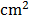### Sample Problem

What is the area of the shaded part of the figure?The area of the shaded part of the figure is.

#### Solution

The area of the rectangle is:

8 × 18 =  144 cm2

The area of the shaded part is half the rectangle

So the area of the shaded part of the figure is:

144 ÷  2 =  72 cm2